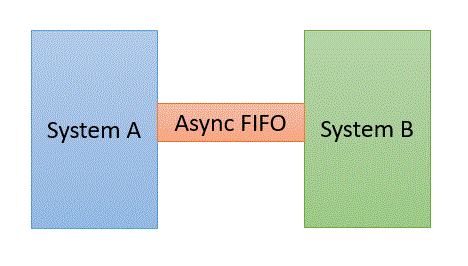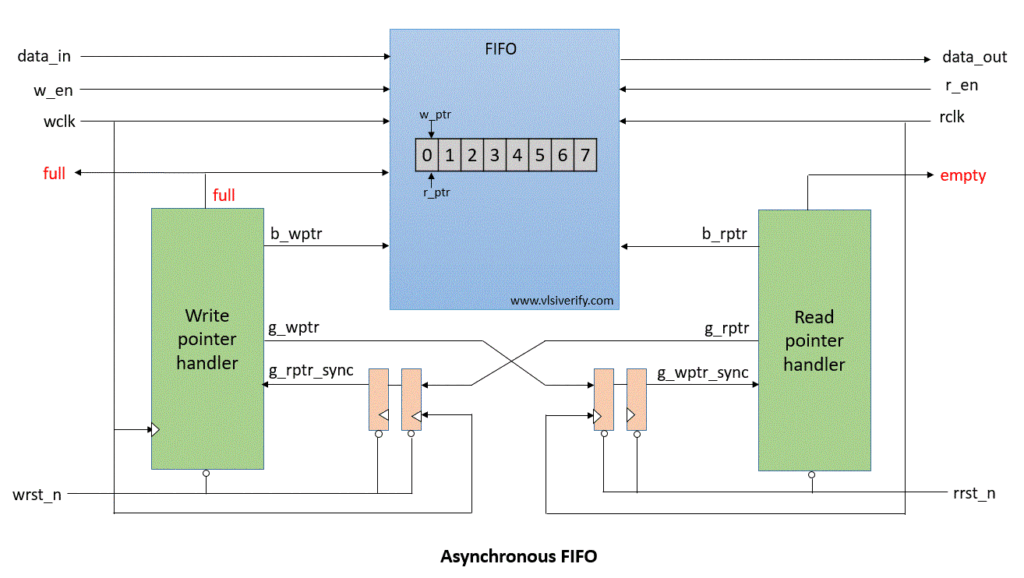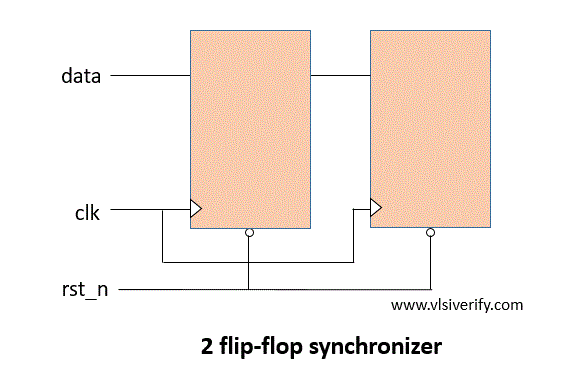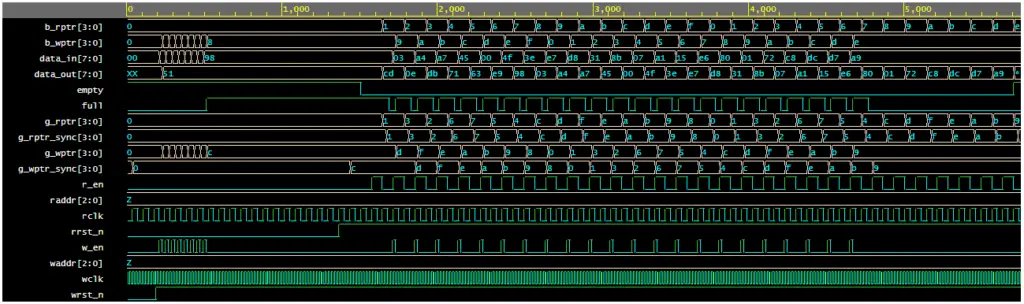Tutorials

In asynchronous FIFO, data read and write operations use different clock frequencies. Since write and read clocks are not synchronized, it is referred to as asynchronous FIFO. Usually, these are used in systems where data need to pass from one clock domain to another which is generally termed as ‘clock domain crossing’. Thus, asynchronous FIFO helps to synchronize data flow between two systems working on different clocks.## Asynchronous FIFO Block DiagramSignals:

wr_en: write enable

wr_data: write data

full: FIFO is full

empty: FIFO is empty

b_wptr: binary write pointer

g_wptr: gray write pointer

b_wptr_next: binary write pointer next

g_wptr_next: gray write pointer next

b_wptr_sync: binary write pointer synchronized

## Asynchronous FIFO Operation

In the case of synchronous FIFO, the write and read pointers are generated on the same clock. However, in the case of asynchronous FIFO write pointer is aligned to the write clock domain whereas the read pointer is aligned to the read clock domain. Hence, it requires domain crossing to calculate FIFO full and empty conditions. This causes metastability in the actual design. In order to resolve this metastability, 2 flip flops or 3 flip flops synchronizer can be used to pass write and read pointers. For explanation, we will go with 2 flip-flop synchronizers. Please note that a single “2 FF synchronizer” can resolve metastability for only one bit. Hence, depending on write and read pointers multiple 2FF synchronizers are required.``````module synchronizer #(parameter WIDTH=3) (input clk, rst_n, [WIDTH:0] d_in, output reg [WIDTH:0] d_out);
reg [WIDTH:0] q1;
always@(posedge clk) begin
if(!rst_n) begin
q1 <= 0;
d_out <= 0;
end
else begin
q1 <= d_in;
d_out <= q1;
end
end
endmodule``````

### Usage of Binary to Gray code converter and vice-versa in Asynchronous FIFO

Till now, we discussed how to get asynchronous write and read pointers in respective clock domains. However, we should not pass binary formatted write and read pointer values. Due to metastability, the overall write or read pointer value might be different.

Example: When binary value wr_ptr = 4’b1101 at the write clock domain is transferred via 2FF synchronizer, at the read clock domain wr_ptr value may receive as 4’b1111 or any other value that is not acceptable. Whereas gray code is assured to have only a single bit change from its previous value. Hence, both write and read pointers need to convert first to their equivalent gray code in their corresponding domain and then pass them to an opposite domain. To check FIFO full and empty conditions in another domain, we have two ways.

#### Way 1

Convert received gray code formatted pointers to binary format and then check for the full and empty conditions.

##### FIFO full condition
``````g2b_converter g2b_wr(g_rptr_sync, b_rptr_sync);
wrap_around = b_rptr_sync[PTR_WIDTH] ^ b_wptr[PTR_WIDTH];
wfull = wrap_around & (b_wptr[PTR_WIDTH-1:0] == b_rptr_sync[PTR_WIDTH-1:0]);``````
##### FIFO empty condition
``````g2b_converter g2b_rd(g_wptr_sync, b_wptr_sync);
rempty = (b_wptr_sync == b_rptr_next);``````

#### Way 2

Check for full and empty conditions directly with the help of gray coded write and read pointer received. This is efficient as it does not need extra hardware for converting gray-coded write and read pointers to equivalent binary forms.

##### FIFO full condition
``wfull = (g_wptr_next == {~g_rptr_sync[PTR_WIDTH:PTR_WIDTH-1], g_rptr_sync[PTR_WIDTH-2:0]});``
##### FIFO empty condition
``rempty = (g_wptr_sync == g_rptr_next);``

## Asynchronous FIFO Verilog Code

### Write Pointer Handler

The output of synchronizer g_rptr_sync is given as an input to ‘write pointer handler’ module used to generate the FIFO full condition. The binary write pointer (b_wptr) is incremented if it satisfies (w_en & !full) condition. This b_wptr value is fed to the fifo_mem module to write data into the FIFO.

``````module wptr_handler #(parameter PTR_WIDTH=3) (
input wclk, wrst_n, w_en,
input [PTR_WIDTH:0] g_rptr_sync,
output reg [PTR_WIDTH:0] b_wptr, g_wptr,
output reg full
);

reg [PTR_WIDTH:0] b_wptr_next;
reg [PTR_WIDTH:0] g_wptr_next;

reg wrap_around;
wire wfull;

assign b_wptr_next = b_wptr+(w_en & !full);
assign g_wptr_next = (b_wptr_next >>1)^b_wptr_next;

always@(posedge wclk or negedge wrst_n) begin
if(!wrst_n) begin
b_wptr <= 0; // set default value
g_wptr <= 0;
end
else begin
b_wptr <= b_wptr_next; // incr binary write pointer
g_wptr <= g_wptr_next; // incr gray write pointer
end
end

always@(posedge wclk or negedge wrst_n) begin
if(!wrst_n) full <= 0;
else        full <= wfull;
end

assign wfull = (g_wptr_next == {~g_rptr_sync[PTR_WIDTH:PTR_WIDTH-1], g_rptr_sync[PTR_WIDTH-2:0]});

endmodule``````

The output of synchronizer g_wptr_sync is given as an input to the ‘read pointer handler’ module to generate FIFO empty condition. The binary read pointer (b_rptr) is incremented if it satisfies (r_en & !empty) condition. This b_rptr value is fed to the fifo_mem module to read data from the FIFO.

``````module rptr_handler #(parameter PTR_WIDTH=3) (
input rclk, rrst_n, r_en,
input [PTR_WIDTH:0] g_wptr_sync,
output reg [PTR_WIDTH:0] b_rptr, g_rptr,
output reg empty
);

reg [PTR_WIDTH:0] b_rptr_next;
reg [PTR_WIDTH:0] g_rptr_next;

assign b_rptr_next = b_rptr+(r_en & !empty);
assign g_rptr_next = (b_rptr_next >>1)^b_rptr_next;
assign rempty = (g_wptr_sync == g_rptr_next);

always@(posedge rclk or negedge rrst_n) begin
if(!rrst_n) begin
b_rptr <= 0;
g_rptr <= 0;
end
else begin
b_rptr <= b_rptr_next;
g_rptr <= g_rptr_next;
end
end

always@(posedge rclk or negedge rrst_n) begin
if(!rrst_n) empty <= 1;
else        empty <= rempty;
end
endmodule``````

### FIFO Memory

Based on binary coded write and read pointers data is written into the FIFO or read from the FIFO respectively.

``````module fifo_mem #(parameter DEPTH=8, DATA_WIDTH=8, PTR_WIDTH=3) (
input wclk, w_en, rclk, r_en,
input [PTR_WIDTH:0] b_wptr, b_rptr,
input [DATA_WIDTH-1:0] data_in,
input full, empty,
output reg [DATA_WIDTH-1:0] data_out
);
reg [DATA_WIDTH-1:0] fifo[0:DEPTH-1];

always@(posedge wclk) begin
if(w_en & !full) begin
fifo[b_wptr[PTR_WIDTH-1:0]] <= data_in;
end
end
/*
always@(posedge rclk) begin
if(r_en & !empty) begin
data_out <= fifo[b_rptr[PTR_WIDTH-1:0]];
end
end
*/
assign data_out = fifo[b_rptr[PTR_WIDTH-1:0]];
endmodule``````

### Top Module

```````include "synchronizer.v"
`include "wptr_handler.v"
`include "rptr_handler.v"
`include "fifo_mem.v"

module asynchronous_fifo #(parameter DEPTH=8, DATA_WIDTH=8) (
input wclk, wrst_n,
input rclk, rrst_n,
input w_en, r_en,
input [DATA_WIDTH-1:0] data_in,
output reg [DATA_WIDTH-1:0] data_out,
output reg full, empty
);

parameter PTR_WIDTH = \$clog2(DEPTH);

reg [PTR_WIDTH:0] g_wptr_sync, g_rptr_sync;
reg [PTR_WIDTH:0] b_wptr, b_rptr;
reg [PTR_WIDTH:0] g_wptr, g_rptr;

synchronizer #(PTR_WIDTH) sync_wptr (rclk, rrst_n, g_wptr, g_wptr_sync); //write pointer to read clock domain
synchronizer #(PTR_WIDTH) sync_rptr (wclk, wrst_n, g_rptr, g_rptr_sync); //read pointer to write clock domain

wptr_handler #(PTR_WIDTH) wptr_h(wclk, wrst_n, w_en,g_rptr_sync,b_wptr,g_wptr,full);
rptr_handler #(PTR_WIDTH) rptr_h(rclk, rrst_n, r_en,g_wptr_sync,b_rptr,g_rptr,empty);
fifo_mem fifom(wclk, w_en, rclk, r_en,b_wptr, b_rptr, data_in,full,empty, data_out);

endmodule``````

## Testbench Code

``````module async_fifo_TB;

parameter DATA_WIDTH = 8;

wire [DATA_WIDTH-1:0] data_out;
wire full;
wire empty;
reg [DATA_WIDTH-1:0] data_in;
reg w_en, wclk, wrst_n;
reg r_en, rclk, rrst_n;

// Queue to push data_in
reg [DATA_WIDTH-1:0] wdata_q[\$], wdata;

asynchronous_fifo as_fifo (wclk, wrst_n,rclk, rrst_n,w_en,r_en,data_in,data_out,full,empty);

always #10ns wclk = ~wclk;
always #35ns rclk = ~rclk;

initial begin
wclk = 1'b0; wrst_n = 1'b0;
w_en = 1'b0;
data_in = 0;

repeat(10) @(posedge wclk);
wrst_n = 1'b1;

repeat(2) begin
for (int i=0; i<30; i++) begin
@(posedge wclk iff !full);
w_en = (i%2 == 0)? 1'b1 : 1'b0;
if (w_en) begin
data_in = \$urandom;
wdata_q.push_back(data_in);
end
end
#50;
end
end

initial begin
rclk = 1'b0; rrst_n = 1'b0;
r_en = 1'b0;

repeat(20) @(posedge rclk);
rrst_n = 1'b1;

repeat(2) begin
for (int i=0; i<30; i++) begin
@(posedge rclk iff !empty);
r_en = (i%2 == 0)? 1'b1 : 1'b0;
if (r_en) begin
wdata = wdata_q.pop_front();
if(data_out !== wdata) \$error("Time = %0t: Comparison Failed: expected wr_data = %h, rd_data = %h", \$time, wdata, data_out);
else \$display("Time = %0t: Comparison Passed: wr_data = %h and rd_data = %h",\$time, wdata, data_out);
end
end
#50;
end

\$finish;
end

initial begin
\$dumpfile("dump.vcd"); \$dumpvars;
end
endmodule
``````

Output:

``````Time = 1575: Comparison Passed: wr_data = 51 and rd_data = 51
Time = 1715: Comparison Passed: wr_data = cd and rd_data = cd
Time = 1855: Comparison Passed: wr_data = 0e and rd_data = 0e
Time = 1995: Comparison Passed: wr_data = db and rd_data = db
Time = 2135: Comparison Passed: wr_data = 71 and rd_data = 71
Time = 2275: Comparison Passed: wr_data = 63 and rd_data = 63
Time = 2415: Comparison Passed: wr_data = e9 and rd_data = e9
Time = 2555: Comparison Passed: wr_data = 98 and rd_data = 98
Time = 2695: Comparison Passed: wr_data = 03 and rd_data = 03
Time = 2835: Comparison Passed: wr_data = a4 and rd_data = a4
Time = 2975: Comparison Passed: wr_data = a7 and rd_data = a7
Time = 3115: Comparison Passed: wr_data = 45 and rd_data = 45
Time = 3255: Comparison Passed: wr_data = 00 and rd_data = 00
Time = 3395: Comparison Passed: wr_data = 4f and rd_data = 4f
Time = 3535: Comparison Passed: wr_data = 3e and rd_data = 3e
Time = 3675: Comparison Passed: wr_data = e7 and rd_data = e7
Time = 3815: Comparison Passed: wr_data = d8 and rd_data = d8
Time = 3955: Comparison Passed: wr_data = 31 and rd_data = 31
Time = 4095: Comparison Passed: wr_data = 8b and rd_data = 8b
Time = 4235: Comparison Passed: wr_data = 07 and rd_data = 07
Time = 4375: Comparison Passed: wr_data = a1 and rd_data = a1
Time = 4515: Comparison Passed: wr_data = 15 and rd_data = 15
Time = 4655: Comparison Passed: wr_data = e6 and rd_data = e6
Time = 4795: Comparison Passed: wr_data = 80 and rd_data = 80
Time = 4935: Comparison Passed: wr_data = 01 and rd_data = 01
Time = 5075: Comparison Passed: wr_data = 72 and rd_data = 72
Time = 5215: Comparison Passed: wr_data = c8 and rd_data = c8
Time = 5355: Comparison Passed: wr_data = dc and rd_data = dc
Time = 5495: Comparison Passed: wr_data = d7 and rd_data = d7
Time = 5635: Comparison Passed: wr_data = a9 and rd_data = a9``````

### WaveformVerilog Codes